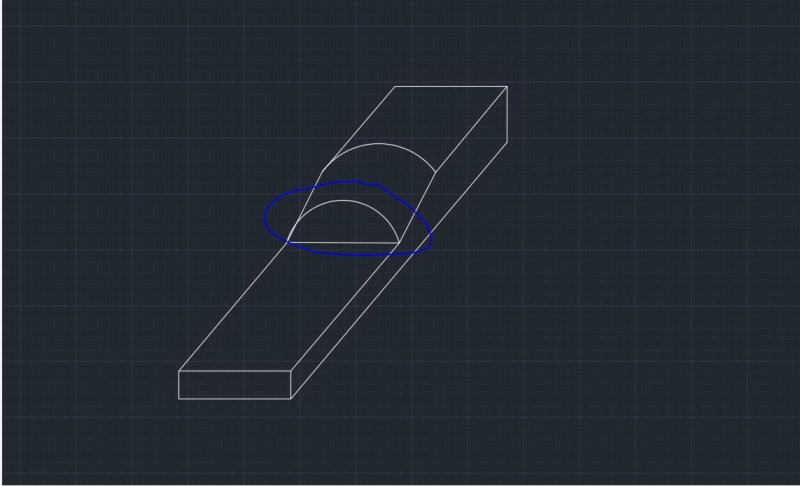# [VBA] How to change angle my Arc## Recommended PostsHi guys,

I have problem, because i dont know how to change angle my arc from 0 to 90 . Any Idea?

```Private Sub CommandButton1_Click()

pi = 4 * Atn(1)

px = 50
py = 50
pz = 0

UserForm1.Hide

b = Val(TextBox2)
c = Val(TextBox3)
d = Val(TextBox4)
e = Val(TextBox5)
f = Val(TextBox6)
g = Val(TextBox7)

Dim r1(0 To 2) As Double
r1(0) = px
r1(1) = py
r1(2) = pz

r2 = ThisDrawing.Utility.PolarPoint(r1, pi, b)
r3 = ThisDrawing.Utility.PolarPoint(r2, 1 / 2 * pi, f + g + e)
r4 = ThisDrawing.Utility.PolarPoint(r3, 0, b)

Dim t1(0 To 2) As Double
t1(0) = px
t1(1) = py
t1(2) = pz + c

With ThisDrawing.Utility
t2 = .PolarPoint(t1, pi, b)
t3 = .PolarPoint(t2, 1 / 2 * pi, f)
t5 = .PolarPoint(t3, 0, b / 2)
t4 = .PolarPoint(t3, 0, b)
End With

With ThisDrawing.ModelSpace

End With

Dim y1(0 To 2) As Double
y1(0) = px
y1(1) = py + f + g
y1(2) = pz + d

With ThisDrawing.Utility
y2 = .PolarPoint(y1, pi, b)
y3 = .PolarPoint(y2, 1 / 2 * pi, e)
y4 = .PolarPoint(y3, 0, b)
End With

With ThisDrawing.ModelSpace
.AddArc t5, b / 2, pi, 0
End With

End Sub```

End Sub## Join the conversation

You can post now and register later. If you have an account, sign in now to post with your account.
Note: Your post will require moderator approval before it will be visible.×   Pasted as rich text.   Restore formatting

Only 75 emoji are allowed.